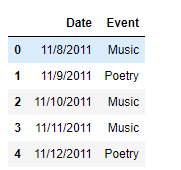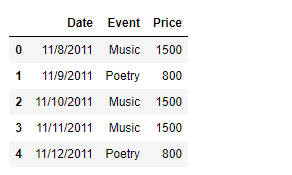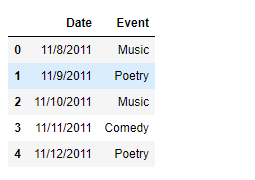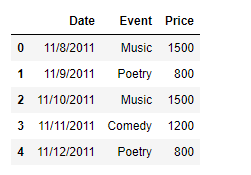# Python | Creating a Pandas dataframe column based on a given condition

• Difficulty Level : Basic
• Last Updated : 21 Jan, 2019

While operating on data, there could be instances where we would like to add a column based on some condition. There does not exist any library function to achieve this task directly, so we are going to see the ways in which we can achieve this goal.

Problem : Given a dataframe containing the data of a cultural event, add a column called ‘Price’ which contains the ticket price for a particular day based on the type of event that will be conducted on that particular day.

Solution #1 : We can use Python’s list comprehension technique to achieve this task. List comprehension is mostly faster than other methods.

 `# importing pandas as pd ` `import` `pandas as pd ` ` `  `# Creating the dataframe ` `df ``=` `pd.DataFrame({``'Date'` `: [``'11/8/2011'``, ``'11/9/2011'``, ``'11/10/2011'``, ` `                                        ``'11/11/2011'``, ``'11/12/2011'``], ` `                ``'Event'` `: [``'Music'``, ``'Poetry'``, ``'Music'``, ``'Music'``, ``'Poetry'``]}) ` ` `  `# Print the dataframe ` `print``(df) `

Output :Now we will add a new column called ‘Price’ to the dataframe. For that purpose, we will use list comprehension technique. Set the price to 1500 if the ‘Event’ is ‘Music’ else 800.

 `# Add a new column named 'Price' ` `df[``'Price'``] ``=` `[``1500` `if` `x ``=``=``'Music'` `else` `800` `for` `x ``in` `df[``'Event'``]] ` ` `  `# Print the DataFrame ` `print``(df) `

Output :As we can see in the output, we have successfully added a new column to the dataframe based on some condition.

Solution #2 : We can use `DataFrame.apply()` function to achieve the goal. There could be instances when we have more than two values, in that case, we can use a dictionary to map new values onto the keys. This does provide a lot of flexibility when we are having a larger number of categories for which we want to assign different values to the newly added column.

 `# importing pandas as pd ` `import` `pandas as pd ` ` `  `# Creating the dataframe ` `df ``=` `pd.DataFrame({``'Date'` `: [``'11/8/2011'``, ``'11/9/2011'``, ``'11/10/2011'``, ` `                                        ``'11/11/2011'``, ``'11/12/2011'``], ` `                ``'Event'` `: [``'Music'``, ``'Poetry'``, ``'Music'``, ``'Comedy'``, ``'Poetry'``]}) ` ` `  `# Print the dataframe ` `print``(df) `

Output :Now we will add a new column called ‘Price’ to the dataframe. For that purpose we will use `DataFrame.apply()` function to achieve the goal. Set the price to 1500 if the ‘Event’ is ‘Music’, 1200 if the ‘Event’ is ‘Comedy’ and 800 if the ‘Event’ is ‘Poetry’.

 `# Define a function to map the values ` `def` `set_value(row_number, assigned_value): ` `    ``return` `assigned_value[row_number] ` ` `  `# Create the dictionary ` `event_dictionary ``=``{``'Music'` `: ``1500``, ``'Poetry'` `: ``800``, ``'Comedy'` `: ``1200``} ` ` `  `# Add a new column named 'Price' ` `df[``'Price'``] ``=` `df[``'Event'``].``apply``(set_value, args ``=``(event_dictionary, )) ` ` `  `# Print the DataFrame ` `print``(df) `

Output :As we can see in the output, we have successfully added a new column to the dataframe based on some condition.

Solution #3 : We can use `DataFrame.map()` function to achieve the goal. It is a very straight forward method where we use a dictionary to simply map values to the newly added column based on the key.

 `# importing pandas as pd ` `import` `pandas as pd ` ` `  `# Creating the dataframe ` `df ``=` `pd.DataFrame({``'Date'` `: [``'11/8/2011'``, ``'11/9/2011'``, ``'11/10/2011'``, ` `                                        ``'11/11/2011'``, ``'11/12/2011'``], ` `                ``'Event'` `: [``'Music'``, ``'Poetry'``, ``'Music'``, ``'Comedy'``, ``'Poetry'``]}) ` ` `  `# Print the dataframe ` `print``(df) `

Output :Now we will add a new column called ‘Price’ to the dataframe. For that purpose we will use `DataFrame.map()` function to achieve the goal. Set the price to 1500 if the ‘Event’ is ‘Music’, 1200 if the ‘Event’ is ‘Comedy’ and 800 if the ‘Event’ is ‘Poetry’.

 `# Create the dictionary ` `event_dictionary ``=``{``'Music'` `: ``1500``, ``'Poetry'` `: ``800``, ``'Comedy'` `: ``1200``} ` ` `  `# Add a new column named 'Price' ` `df[``'Price'``] ``=` `df[``'Event'``].``map``(event_dictionary) ` ` `  `# Print the DataFrame ` `print``(df) `

Output :My Personal Notes arrow_drop_up
Recommended Articles
Page :